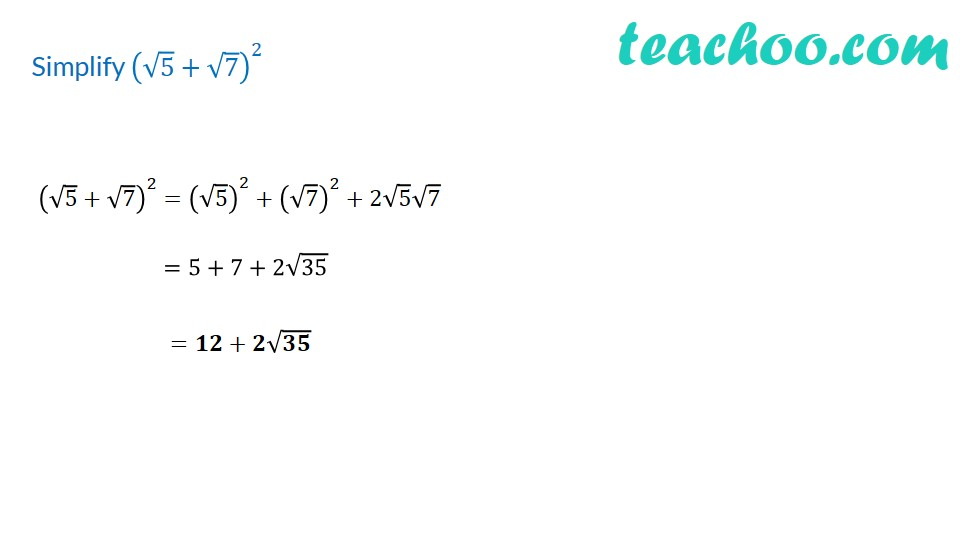1. Chapter 1 Class 9 Number Systems (Term 1)
2. Concept wise
3. Rationalising

Transcript

Simplify (√5+√7)^2 (√5+√7)^2 =5+7+2√35 =𝟏𝟐+𝟐√𝟑𝟓 =(√5)^2+(√7)^2+2√5 √7